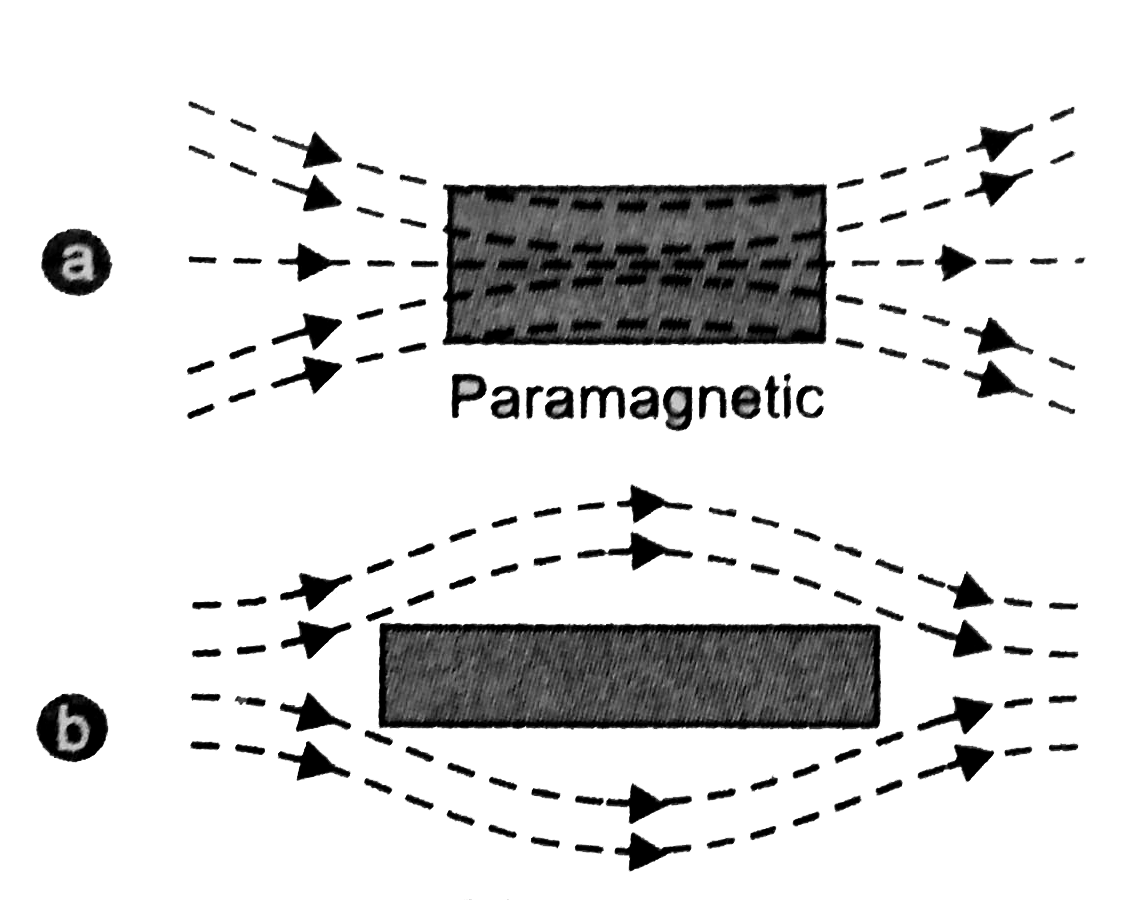# Paramagnetic materials | definition, properties, and examples, class 12

Knowledge Increases By Sharing...

In this article, we will discuss Paramagnetism, and paramagnetic materials, their definition, properties, and class 12, so let’s get started.

Inside Story

## What is paramagnetism?

Paramagnetism is a form of magnetism in which some materials are weakly attracted towards an externally applied magnetic field, and form internally, induced magnetic fields in the direction of the applied magnetic field.

But, contrary to this behavior, diamagnetic materials are those, which are repelled by magnetic fields and form induced magnetic fields in the direction, opposite to that of the applied magnetic field. Most of the chemical elements and some compounds are materials that possess paramagnetic properties.

Paramagnetic materials have a relative magnetic permeability slightly greater than 1 (means, it has a small positive magnetic susceptibility) and hence, they are attracted towards magnetic fields.

The magnetic moment induced by the applied magnetic field is linear in field strength and is fairly weak. A sensitive analytical balance is usually required to demonstrate the effect, and modern measurements on paramagnetic materials are often made with a SQUID magnetometer.[latexpage]

## What is paramagnetic materials?

Paramagnetic materials are materials that tend to get weakly magnetized in the direction of the magnetizing field when they are placed in a magnetic field.

Paramagnetic materials have a permanent dipole moment or permanent magnetic moment. However, unlike ferromagnetism, it does not retain any magnetization in the absence of an externally applied magnetic field. If we remove the applied magnetic field the materials tend to lose their magnetism. This is because the thermal motion randomizes the spin orientations of the electrons.

When paramagnets are placed in the magnetic field then, there is a little amount of magnetization is induced in it, because only a small fraction of the spins are oriented by the field. This fraction is proportional to the intensity of the field and this explains the linear dependence.

The attraction that ferromagnetic materials experience is non-linear and much stronger than that of paramagnets, so it is easily observed in the attraction between a fridge magnet and the iron in the fridge itself.

## What causes paramagnetism?

Paramagnetism is caused due to the presence of unpaired electrons in the material, if the atomic orbitals of most of the atoms are incomplete then they are all paramagnetic in nature, but there are exceptions such as copper. Due to their spin, unpaired electrons have a magnetic dipole moment and act like small magnets.

The external magnetic field causes the electron spins to align parallel to the field, creating a net attraction. Paramagnetic materials include aluminum, oxygen, titanium, and iron oxide (FeO). Therefore, in chemistry, a simple rule of thumb is used to determine whether a particle (atom, ion, or molecule) is paramagnetic or diamagnetic.

If all the electrons in the particle are paired, then the substance formed by that particle is diamagnetic; if it has unpaired electrons, then the substance is paramagnetic.

## Properties of paramagnetic materials

Some properties of paramagnetic materials are given below:

• When paramagnetic materials are placed in external magnetic field, it develops feeble magnetization in the direction of the applied field.
• When a rod made up of paramagnetic materials in kept in the external magnetic field, then the line of force i.e magnetic fields prefer to pass through the rod than the surrounding air. In othrs words, lines of forces get more concentrated inside the rod materials, see figure below. The magnetic induction B becomes slightly greater than the magnetising field, $B_0=\mu_0H$. This increase is very small, about 1 part in $10^5$.a). Slightly concentrated magnetic lines of force in paramagnetic rod. b) magnetic field around a diamagnetic materials, source: doubtnut
• When a paramagnetic materials is placed in non-uniform magnetic field, it tends to moves from weaker to the stronger parts of the magnetic fields.
• When a paramagnetic rod is suspended freely in a uniform magntic field, it tries to aligns itself para;llel to the magnetizing field.
• A paramagnetic liquid in a U-Tube ascends in the limb which is between the poles of the magnet.Intensity of magnetization is proportional to the magnetizing field, source: esaral
• A paramagnetic materials develops small magnetization in the direction of the magnetizing field, so its susceptibility has small but positive value. For aluminium, $\chi=1.8\times 10^{-6}$.
• The relative permeability $(\mu_r = 1+\chi_m)$ for a paramagnetic materials slightly greater than 1.
• The magnetic susceptibility of paramagnetic materials varies inversly to their absolute temperature T, i.e $$\chi_m \propto\frac{1}{T}$$ $$or\qquad \chi_m =\frac{C}{T}$$ where C is a constant called Curie’s constant and this equation is known as Curie’s law.
• For a given temperature, the intensity of magnetization is proportional to the magnetizing field, so the susceptibility and permeability do not show any variation with the field $\vec{B_0}$.
• As soon as the magnetic field is removed, paramagnetic materials start loosing its magnetism.

## Paramagnetic liquid demonstration

When a watch glass containing a paramagnetic liquid is placed over two closely lying two magnetic poles, the liquid accumulates and elevates in the middle and becomes thin near the poles. This is because the field in the centre is the strongest.Elevation of paramagnetic liquid, when placed over two closely lying two magnetic pole, source: esaral

When the poles are apart, the field at the poles becomes stronger than that at the centre and the liquid moves toward the poles. This creates a depression in the middle because the field is strongest at poles.Depression of paramagnetic liquid, when placed over two distant lying two magnetic pole, source: esaral

## Supermagnets

Superparamagnets are the materials that not only shows a net paramagnetic response, but also tend to shows strong ferromagnetic or ferrimagnetic ordering mainly at the microscopic level. These materials also follow Curie’s law and have very large Curie constants. Ferrofluids are a example superparamagnetic.

## Examples of paramagnetic materials

Some of the examples of paramagnetic materials include iron oxide, oxygen, titanium, aluminum, transition metal complexes, etc.

YesNo
Knowledge Increases By Sharing...